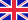•# Some Problems on a Class of Fluid Dynamical Systems

Autor: Manwai Yuen

In this monograph, we consider the solutions for a class of fluid dynamical systems, such as the isentropic Euler-Poisson, Navier-Stokes-Poisson, Navier-Stokes, Euler equations. As these systems share some similar mathematical structures, we would like... Viac o knihe

Na objednávku, dodanie 2-4 týždne

42.84 €

bežná cena: 50.40 €

## O knihe

In this monograph, we consider the solutions for a class of fluid dynamical systems, such as the isentropic Euler-Poisson, Navier-Stokes-Poisson, Navier-Stokes, Euler equations. As these systems share some similar mathematical structures, we would like to find the relationship between them, such as some blowup and stability phenomena. We have constructed the blowup (collapsing) solutions for the Euler-Poisson and Navier-Stokes-Poisson systems and the systems with frictional damping. In particular, the analytical blowup solutions for the 2 dimensional isothermal (=1) systems are the core result. Then, the blowup rates of the solutions are studied. The special solutions for the constant pressure systems are also covered. And some special blowup solutions for these systems in radially symmetry are given. After that, the general existence of blowup solutions for the Euler system with constant pressure is obtained. In addition, we present a special blowup solution for the Euler system. The stabilities of the Euler-Poisson equations are also considered. Some open problems are discussed on the 3 dimensional Euler-Poisson equations in the end.

• Vydavateľstvo: VDM Verlag
• Rok vydania: 2009
• Formát: Paperback
• Rozmer: 220 x 150 mm
• Jazyk: Anglický jazyk
• ISBN: 9783639183573

Generuje redakčný systém BUXUS CMS spoločnosti ui42.